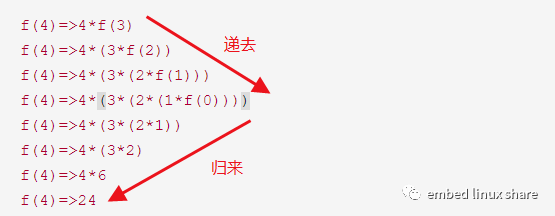# 为什么说递归是码农的一道分水岭?

• 不能理解递归，意味着归并、快排、二叉树、回溯、贪心、动态规划等人类花了几十数百年总结出来的这些优秀算法，对你而言只是个躺在书本上毫无价值的死代码。

• 不能理解递归，意味着编译原理的词法分析、语法分析等，你将一无所知。虽然大部分人都没机会接触编译器底层机制，但是天天使用着各种各样的编译器，却对它内部一无所知，是个小遗憾。

• 不能理解递归，意味着在大规模复杂情景下的开发，不得不忍受迭代的繁琐，以及无比臃肿丑陋的代码。

# 递归的哲学

古之欲明明德于天下者，



# 一个例子

int f(int n)
{
int sum = 0;
if (0 == n)
return 1;
else
sum = n * f(n-1);
return sum;
}


f(4)=>4*f(3)
f(4)=>4*(3*f(2))
f(4)=>4*(3*(2*f(1)))
f(4)=>4*(3*(2*(1*f(0))))
f(4)=>4*(3*(2*1))
f(4)=>4*(3*2)
f(4)=>4*6
f(4)=>24function recursion(大规模){
if (end_condition){      // 明确的递归终止条件
end;   // 简单情景
}else{            // 先将问题全部描述展开，再由尽头“返回”依次解决每步中剩余部分的问题
recursion(小规模);     // 递去
solve;                // 归来
}
}©️2019 CSDN 皮肤主题: 编程工作室 设计师: CSDN官方博客